Скачать презентацию FILTRATION Separation of solids from liquids by

• Количество слайдов: 26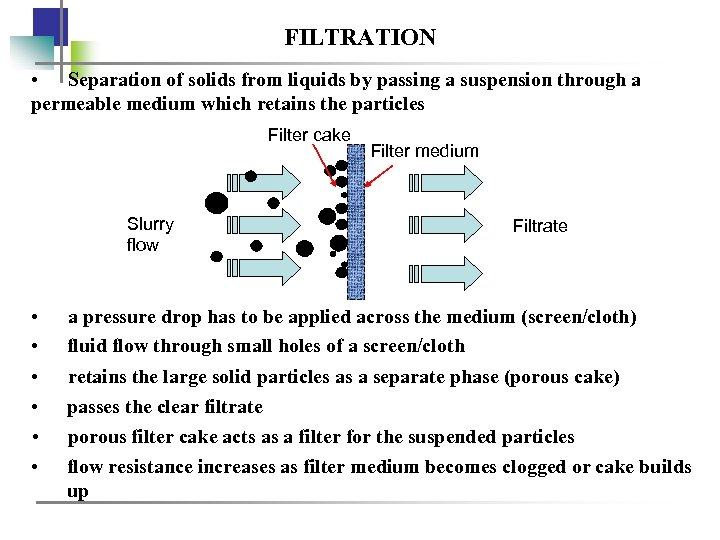FILTRATION • Separation of solids from liquids by passing a suspension through a permeable medium which retains the particles Filter cake Slurry flow Filter medium Filtrate • • a pressure drop has to be applied across the medium (screen/cloth) fluid flow through small holes of a screen/cloth • • retains the large solid particles as a separate phase (porous cake) passes the clear filtrate porous filter cake acts as a filter for the suspended particles flow resistance increases as filter medium becomes clogged or cake builds up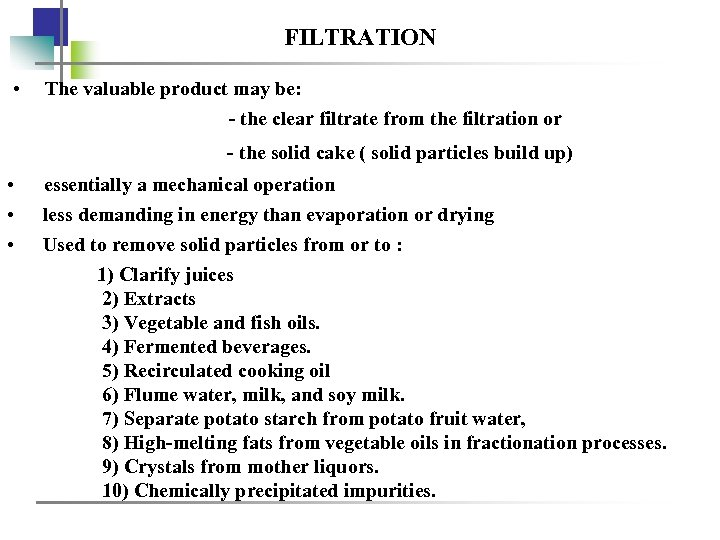FILTRATION • The valuable product may be: - the clear filtrate from the filtration or - the solid cake ( solid particles build up) • • • essentially a mechanical operation less demanding in energy than evaporation or drying Used to remove solid particles from or to : 1) Clarify juices 2) Extracts 3) Vegetable and fish oils. 4) Fermented beverages. 5) Recirculated cooking oil 6) Flume water, milk, and soy milk. 7) Separate potato starch from potato fruit water, 8) High-melting fats from vegetable oils in fractionation processes. 9) Crystals from mother liquors. 10) Chemically precipitated impurities.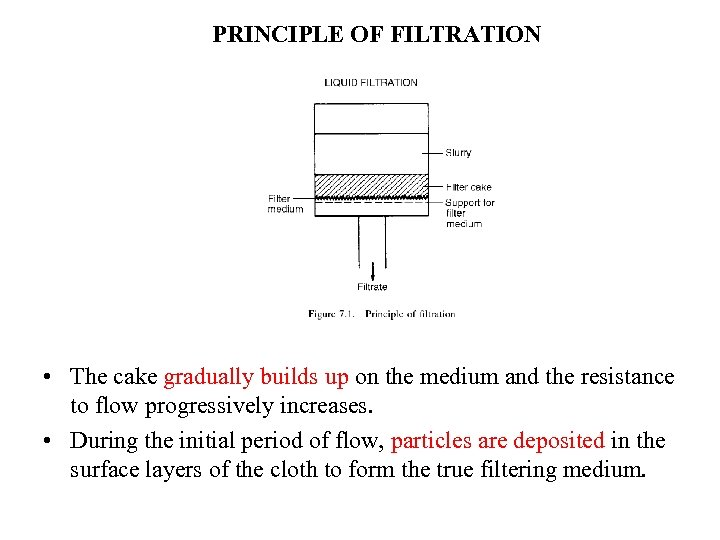PRINCIPLE OF FILTRATION • The cake gradually builds up on the medium and the resistance to flow progressively increases. • During the initial period of flow, particles are deposited in the surface layers of the cloth to form the true filtering medium.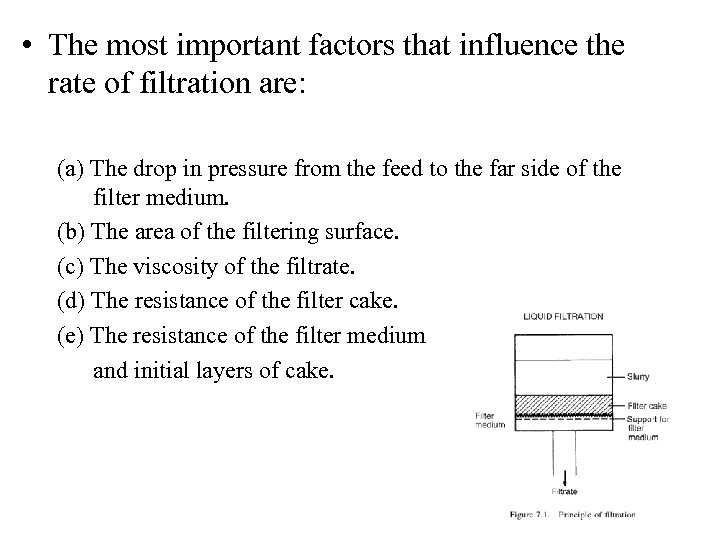• The most important factors that influence the rate of filtration are: (a) The drop in pressure from the feed to the far side of the filter medium. (b) The area of the filtering surface. (c) The viscosity of the filtrate. (d) The resistance of the filter cake. (e) The resistance of the filter medium and initial layers of cake.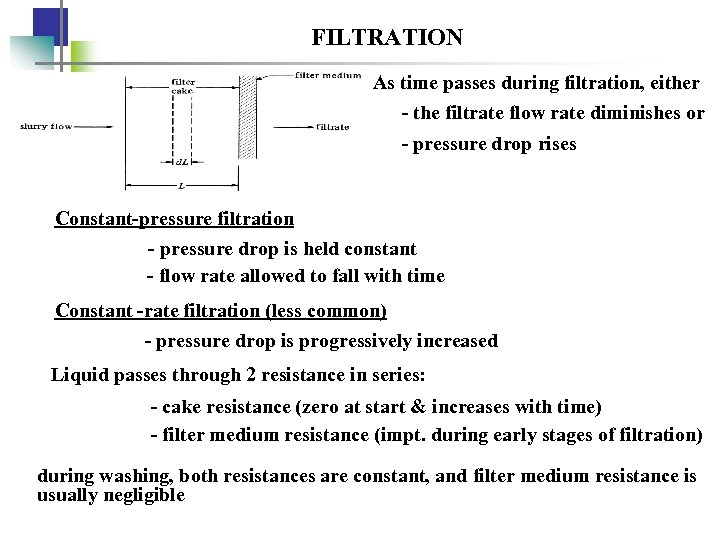FILTRATION As time passes during filtration, either - the filtrate flow rate diminishes or - pressure drop rises Constant-pressure filtration - pressure drop is held constant - flow rate allowed to fall with time Constant -rate filtration (less common) - pressure drop is progressively increased Liquid passes through 2 resistance in series: - cake resistance (zero at start & increases with time) - filter medium resistance (impt. during early stages of filtration) during washing, both resistances are constant, and filter medium resistance is usually negligible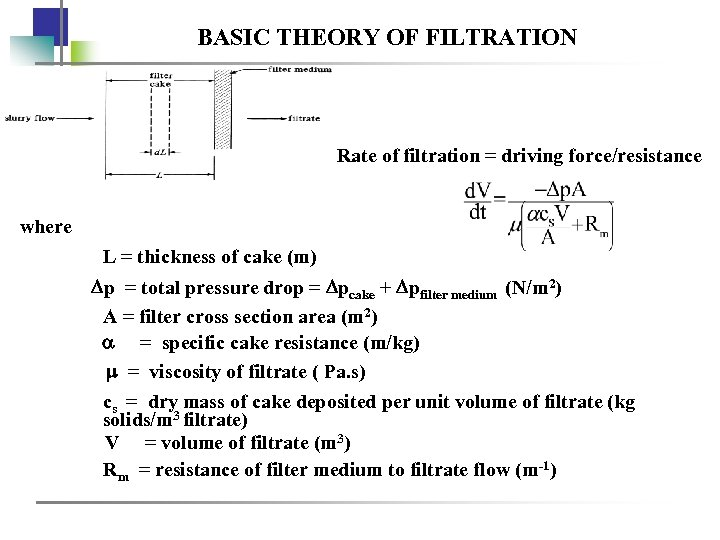BASIC THEORY OF FILTRATION Rate of filtration = driving force/resistance where L = thickness of cake (m) p = total pressure drop = pcake + pfilter medium (N/m 2) A = filter cross section area (m 2) = specific cake resistance (m/kg) = viscosity of filtrate ( Pa. s) cs = dry mass of cake deposited per unit volume of filtrate (kg solids/m 3 filtrate) V = volume of filtrate (m 3) Rm = resistance of filter medium to filtrate flow (m-1)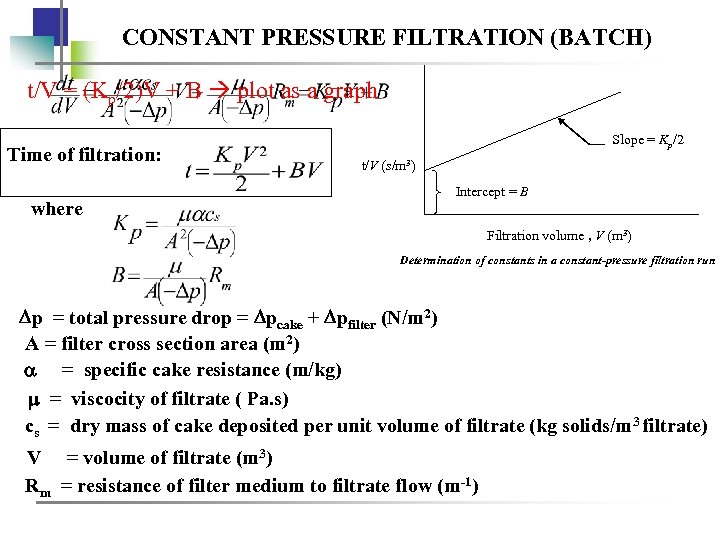CONSTANT PRESSURE FILTRATION (BATCH) t/V = (Kp/2)V + B plot as a graph Time of filtration: where Slope = Kp/2 t/V (s/m 3) Intercept = B Filtration volume , V (m 3) Determination of constants in a constant-pressure filtration run p = total pressure drop = pcake + pfilter (N/m 2) A = filter cross section area (m 2) = specific cake resistance (m/kg) = viscocity of filtrate ( Pa. s) cs = dry mass of cake deposited per unit volume of filtrate (kg solids/m 3 filtrate) V = volume of filtrate (m 3) Rm = resistance of filter medium to filtrate flow (m-1)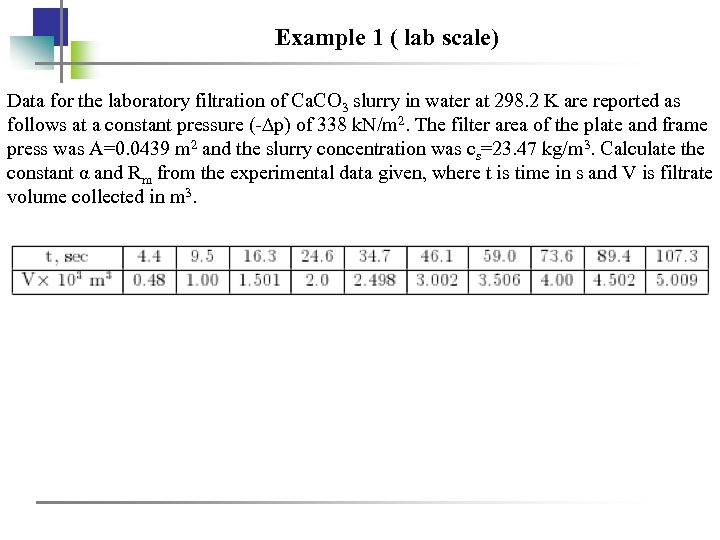Example 1 ( lab scale) Data for the laboratory filtration of Ca. CO 3 slurry in water at 298. 2 K are reported as follows at a constant pressure (-∆p) of 338 k. N/m 2. The filter area of the plate and frame press was A=0. 0439 m 2 and the slurry concentration was cs=23. 47 kg/m 3. Calculate the constant α and Rm from the experimental data given, where t is time in s and V is filtrate volume collected in m 3.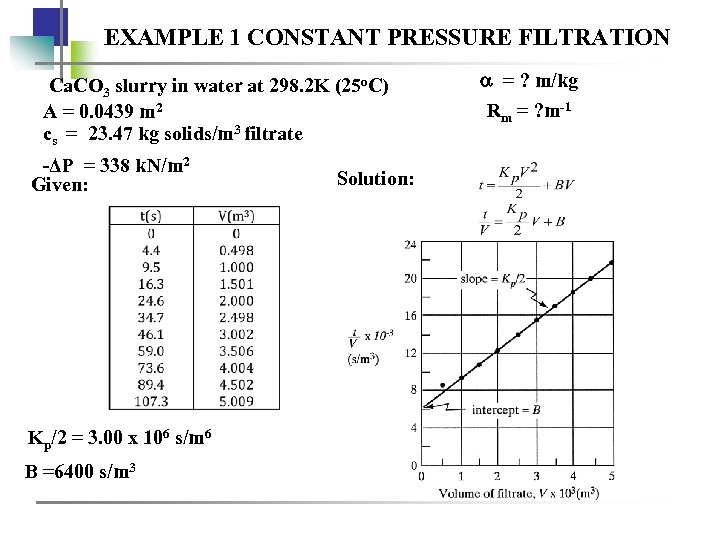EXAMPLE 1 CONSTANT PRESSURE FILTRATION Ca. CO 3 slurry in water at 298. 2 K (25 o. C) A = 0. 0439 m 2 cs = 23. 47 kg solids/m 3 filtrate -ΔP = 338 k. N/m 2 Given: Kp/2 = 3. 00 x 106 s/m 6 B =6400 s/m 3 Solution: = ? m/kg Rm = ? m-1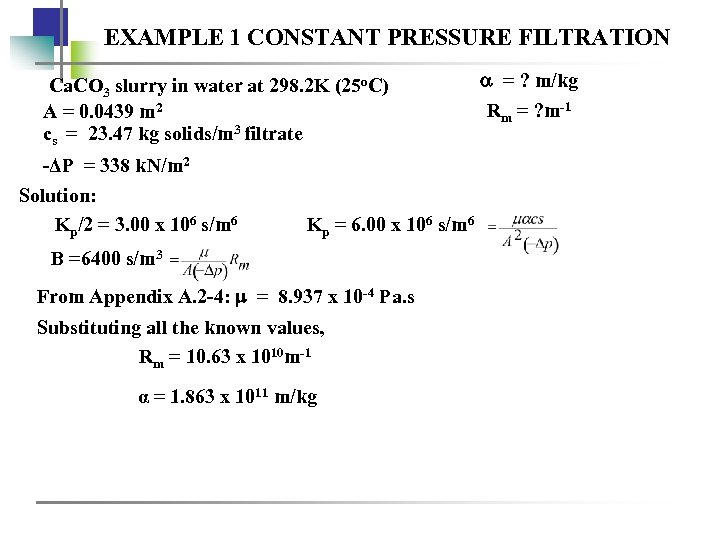EXAMPLE 1 CONSTANT PRESSURE FILTRATION Ca. CO 3 slurry in water at 298. 2 K (25 o. C) A = 0. 0439 m 2 cs = 23. 47 kg solids/m 3 filtrate -ΔP = 338 k. N/m 2 Solution: Kp/2 = 3. 00 x 106 s/m 6 Kp = 6. 00 x 106 s/m 6 B =6400 s/m 3 From Appendix A. 2 -4: = 8. 937 x 10 -4 Pa. s Substituting all the known values, Rm = 10. 63 x 1010 m-1 α = 1. 863 x 1011 m/kg = ? m/kg Rm = ? m-1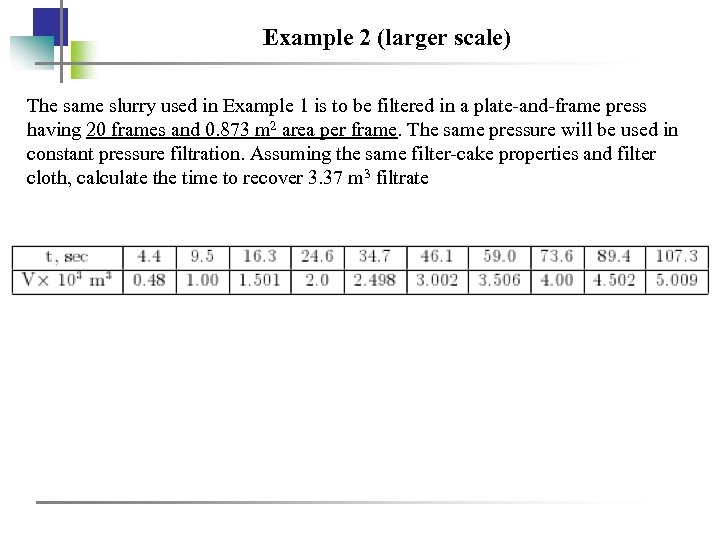Example 2 (larger scale) The same slurry used in Example 1 is to be filtered in a plate-and-frame press having 20 frames and 0. 873 m 2 area per frame. The same pressure will be used in constant pressure filtration. Assuming the same filter-cake properties and filter cloth, calculate the time to recover 3. 37 m 3 filtrate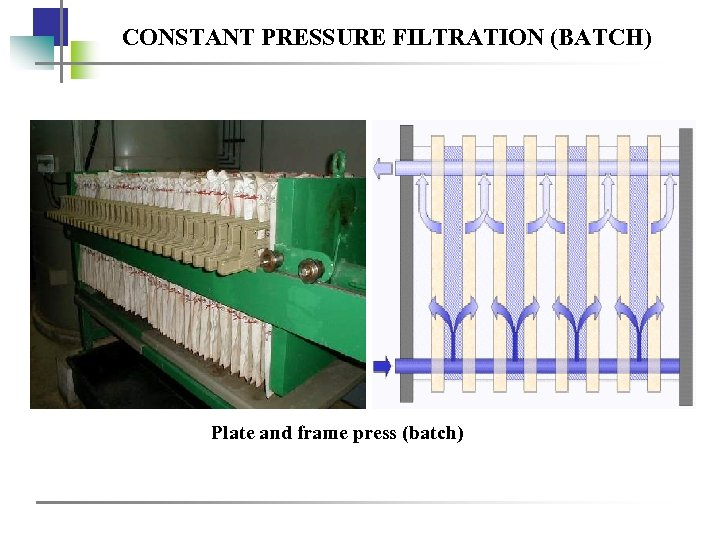CONSTANT PRESSURE FILTRATION (BATCH) Plate and frame press (batch)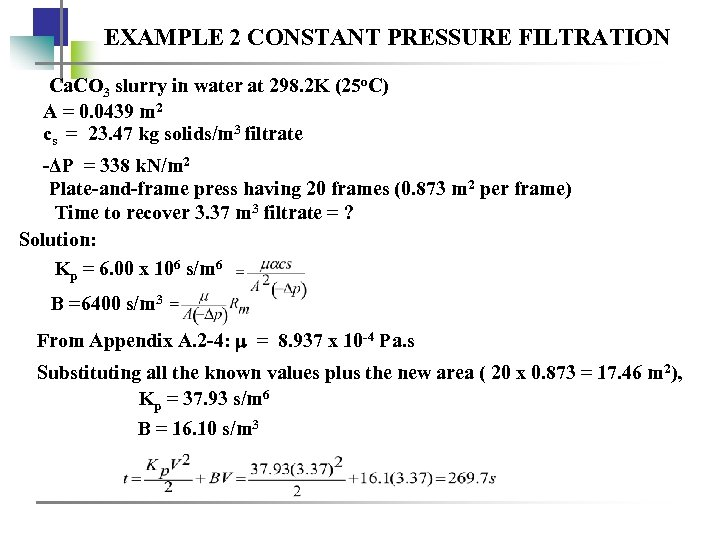EXAMPLE 2 CONSTANT PRESSURE FILTRATION Ca. CO 3 slurry in water at 298. 2 K (25 o. C) A = 0. 0439 m 2 cs = 23. 47 kg solids/m 3 filtrate -ΔP = 338 k. N/m 2 Plate-and-frame press having 20 frames (0. 873 m 2 per frame) Time to recover 3. 37 m 3 filtrate = ? Solution: Kp = 6. 00 x 106 s/m 6 B =6400 s/m 3 From Appendix A. 2 -4: = 8. 937 x 10 -4 Pa. s Substituting all the known values plus the new area ( 20 x 0. 873 = 17. 46 m 2), Kp = 37. 93 s/m 6 B = 16. 10 s/m 3CONSTANT PRESSURE FILTRATION (BATCH) Plate and frame press (batch)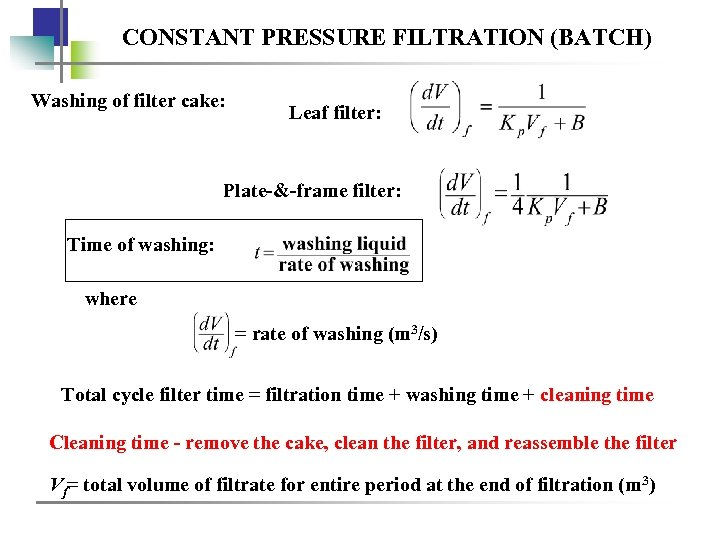CONSTANT PRESSURE FILTRATION (BATCH) Washing of filter cake: Leaf filter: Plate-&-frame filter: Time of washing: where = rate of washing (m 3/s) Total cycle filter time = filtration time + washing time + cleaning time Cleaning time - remove the cake, clean the filter, and reassemble the filter Vf= total volume of filtrate for entire period at the end of filtration (m 3)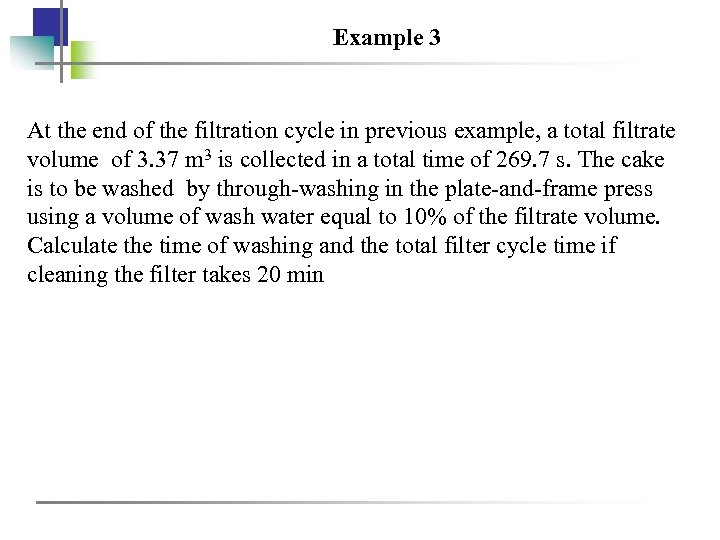Example 3 At the end of the filtration cycle in previous example, a total filtrate volume of 3. 37 m 3 is collected in a total time of 269. 7 s. The cake is to be washed by through-washing in the plate-and-frame press using a volume of wash water equal to 10% of the filtrate volume. Calculate the time of washing and the total filter cycle time if cleaning the filter takes 20 min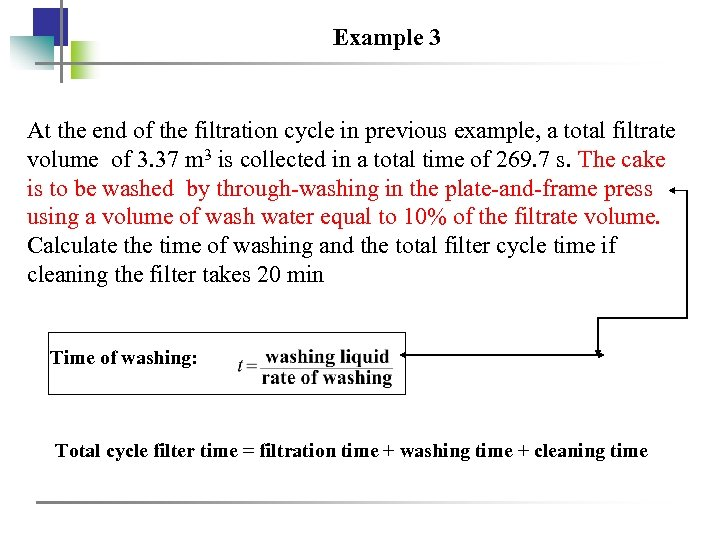Example 3 At the end of the filtration cycle in previous example, a total filtrate volume of 3. 37 m 3 is collected in a total time of 269. 7 s. The cake is to be washed by through-washing in the plate-and-frame press using a volume of wash water equal to 10% of the filtrate volume. Calculate the time of washing and the total filter cycle time if cleaning the filter takes 20 min Time of washing: Total cycle filter time = filtration time + washing time + cleaning time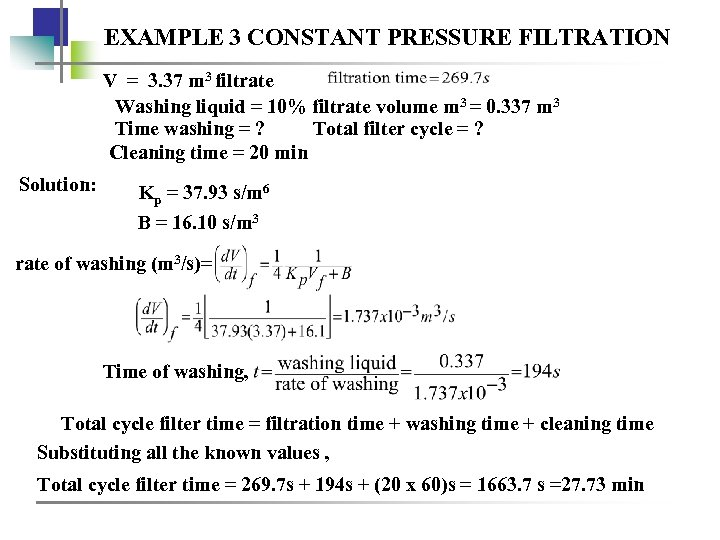EXAMPLE 3 CONSTANT PRESSURE FILTRATION V = 3. 37 m 3 filtrate Washing liquid = 10% filtrate volume m 3 = 0. 337 m 3 Time washing = ? Total filter cycle = ? Cleaning time = 20 min Solution: Kp = 37. 93 s/m 6 B = 16. 10 s/m 3 rate of washing (m 3/s)= Time of washing, Total cycle filter time = filtration time + washing time + cleaning time Substituting all the known values , Total cycle filter time = 269. 7 s + 194 s + (20 x 60)s = 1663. 7 s =27. 73 minCONSTANT PRESSURE FILTRATION (BATCH) Plate and frame press (batch)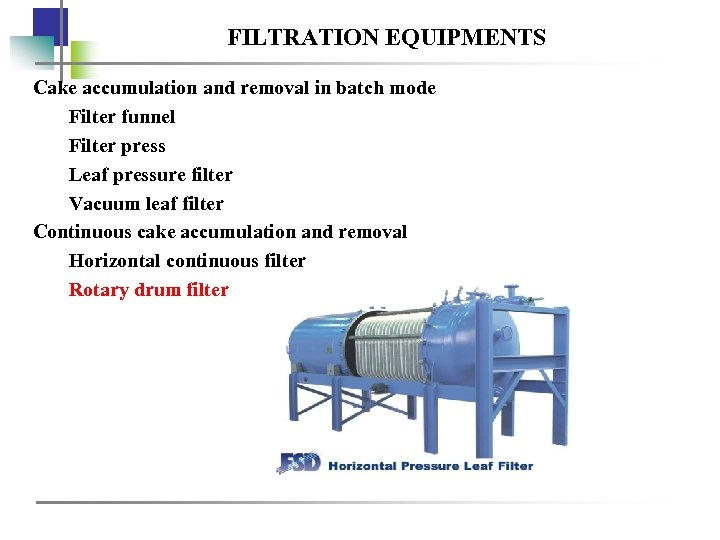FILTRATION EQUIPMENTS Cake accumulation and removal in batch mode Filter funnel Filter press Leaf pressure filter Vacuum leaf filter Continuous cake accumulation and removal Horizontal continuous filter Rotary drum filter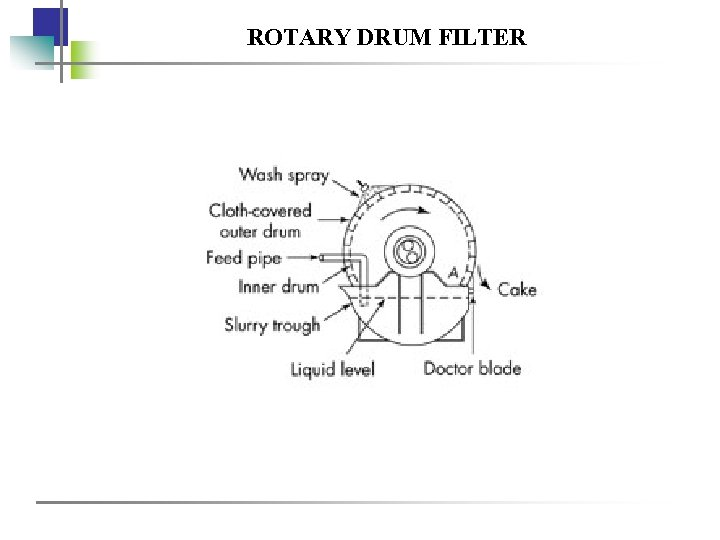ROTARY DRUM FILTER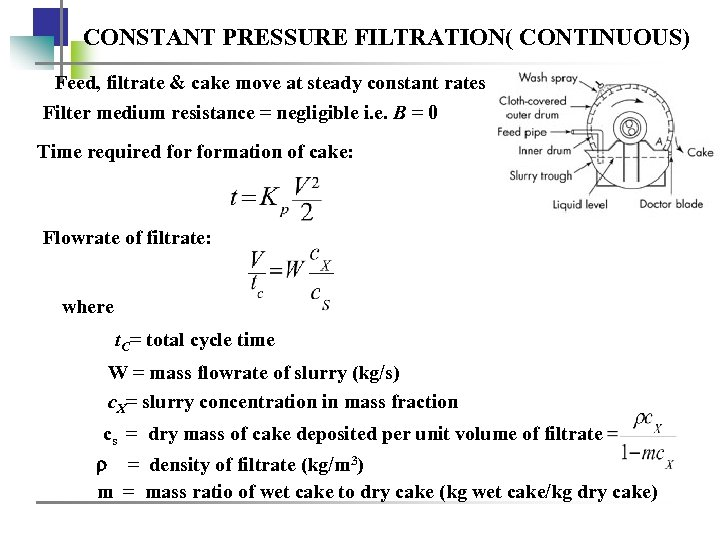CONSTANT PRESSURE FILTRATION( CONTINUOUS) Feed, filtrate & cake move at steady constant rates Filter medium resistance = negligible i. e. B = 0 Time required formation of cake: Flowrate of filtrate: where t. C= total cycle time W = mass flowrate of slurry (kg/s) c. X= slurry concentration in mass fraction cs = dry mass of cake deposited per unit volume of filtrate = density of filtrate (kg/m 3) m = mass ratio of wet cake to dry cake (kg wet cake/kg dry cake)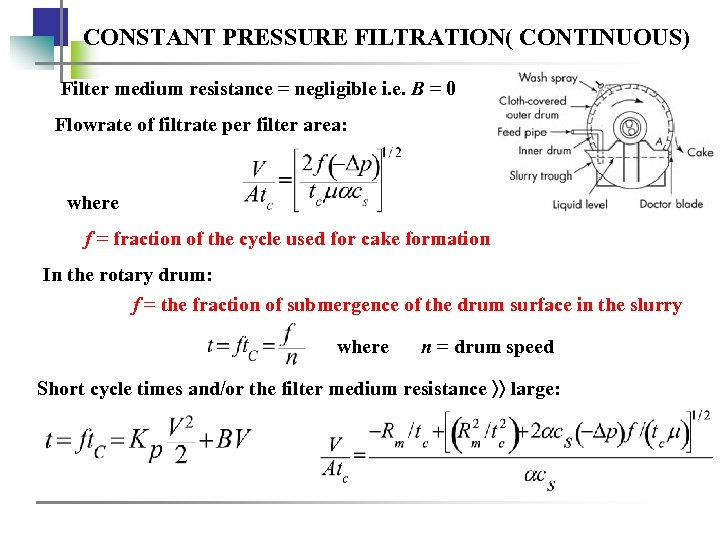CONSTANT PRESSURE FILTRATION( CONTINUOUS) Filter medium resistance = negligible i. e. B = 0 Flowrate of filtrate per filter area: where f = fraction of the cycle used for cake formation In the rotary drum: f = the fraction of submergence of the drum surface in the slurry where n = drum speed Short cycle times and/or the filter medium resistance large: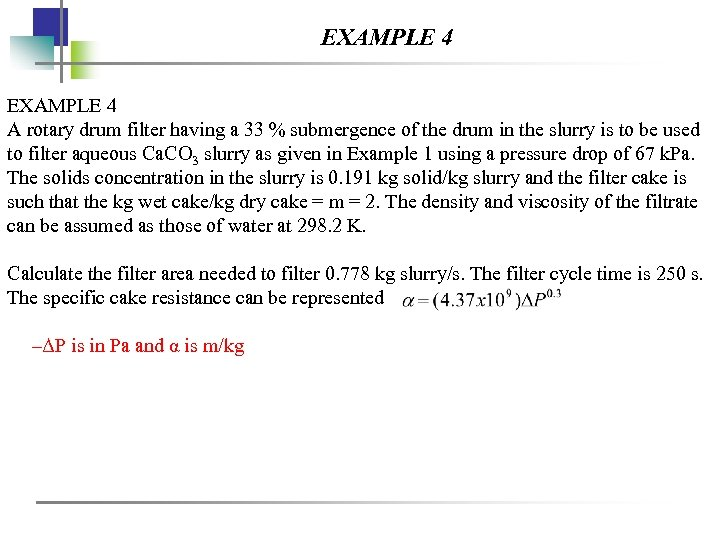EXAMPLE 4 A rotary drum filter having a 33 % submergence of the drum in the slurry is to be used to filter aqueous Ca. CO 3 slurry as given in Example 1 using a pressure drop of 67 k. Pa. The solids concentration in the slurry is 0. 191 kg solid/kg slurry and the filter cake is such that the kg wet cake/kg dry cake = m = 2. The density and viscosity of the filtrate can be assumed as those of water at 298. 2 K. Calculate the filter area needed to filter 0. 778 kg slurry/s. The filter cycle time is 250 s. The specific cake resistance can be represented –ΔP is in Pa and α is m/kg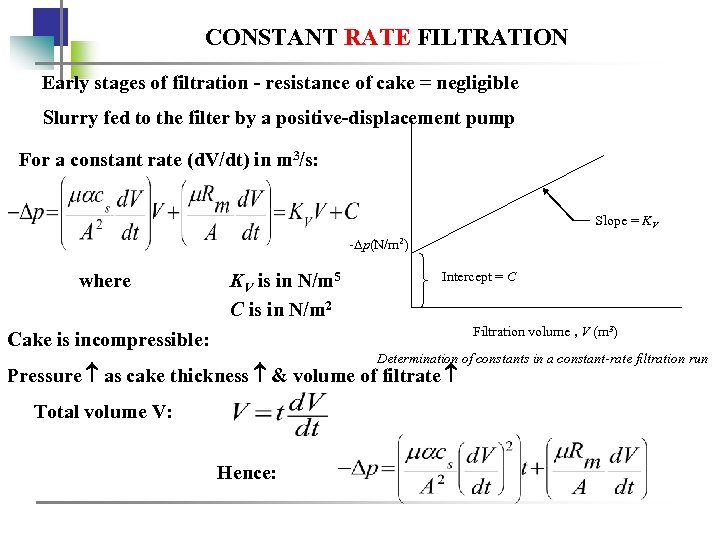CONSTANT RATE FILTRATION Early stages of filtration - resistance of cake = negligible Slurry fed to the filter by a positive-displacement pump For a constant rate (d. V/dt) in m 3/s: Slope = KV - p(N/m 2) where KV is in N/m 5 C is in N/m 2 Intercept = C Filtration volume , V (m 3) Cake is incompressible: Determination of constants in a constant-rate filtration run Pressure as cake thickness & volume of filtrate Total volume V: Hence: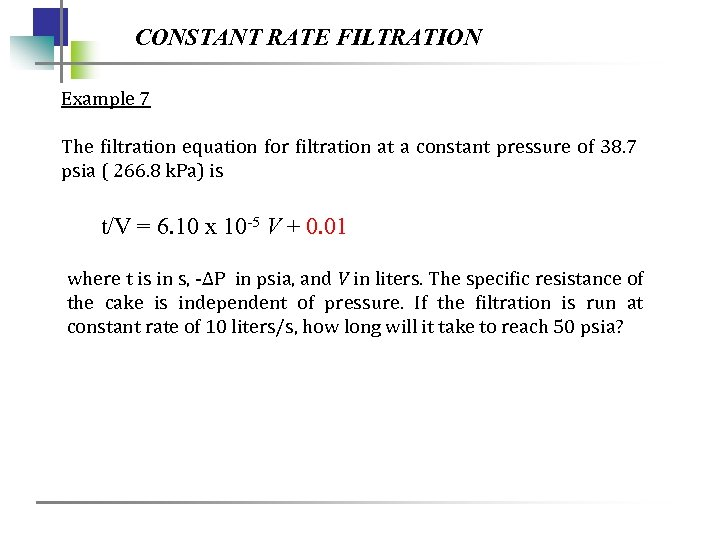CONSTANT RATE FILTRATION Example 7 The filtration equation for filtration at a constant pressure of 38. 7 psia ( 266. 8 k. Pa) is t/V = 6. 10 x 10 -5 V + 0. 01 where t is in s, -ΔP in psia, and V in liters. The specific resistance of the cake is independent of pressure. If the filtration is run at constant rate of 10 liters/s, how long will it take to reach 50 psia?